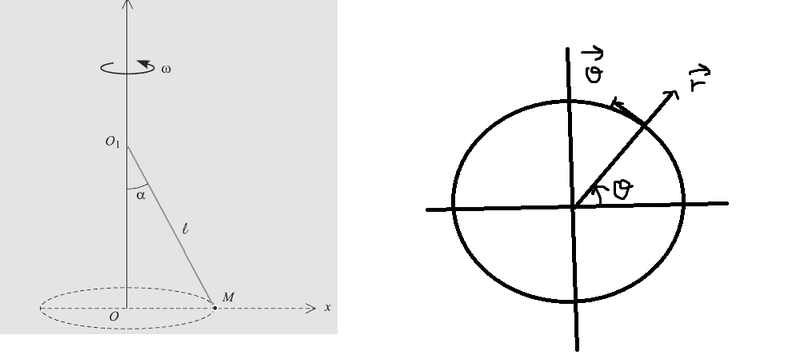# Why they don't give me the same answer? dynamics of uniform circular motion

• Andrax
In summary: Physics Forums for a few days now. He has been told to use a free body diagram, but he is not sure how to do it. He is also not sure what the question is.

## Homework Statement

So a body $m$ is on a uniform circular motion ($\omega = d\theta/dt = \text{constant}$), it is suspended by an inextensible rope with negligeable mass## Homework Equations

P = mg , didnt know what to put here

## The Attempt at a Solution

So this is what the book did.

What I did I drew the same diagram on another way so I an use polar coordinates (second picture)

So the Weight is perpendicular to the vector $r$ and $\theta$, this is a problem since we won't have $mg$ in our equation at the end like the reasoning with the other picture but let's continue. On r:

$$- T \sin \alpha = -rw^2$$

on $\theta$:

$$-T \cos \alpha = 0$$

And this is wrong.

I've been confused for 3 days in a row , what am I doing wrong?

: http://i.stack.imgur.com/FntS7.png
[/B]

Second picture is he same system but from uppoint of view , it gives me diffrent results

I did not see explained what you were trying to determine. What is the question?

You need a good free-body diagram. You have weight of the mass. You know it is a constant omega, so you know that at any given moment it has an acceleration towards the centre of the circle. The mass is accelerating so at any moment there is a net force on it. What direction does the net force on the mass point? And what will be the magnitude of this net force on the mass? What is the tension in the string?